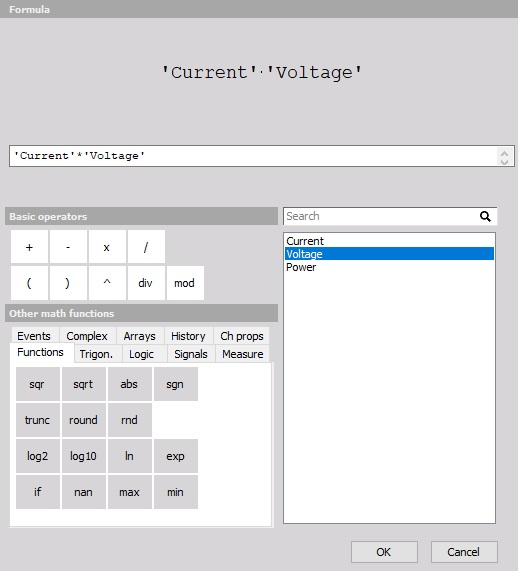# Setup screen and basic operation

When you choose any of Dewesoft Math setup screen buttons with specific Math function (e.g. formula button), a new Math channel is displayed: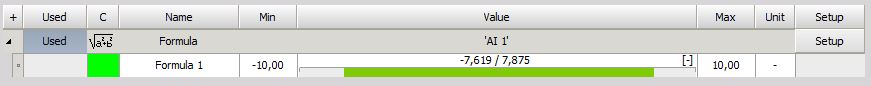The columns in the new Math line look similar to the analog channel setup:

• Used/Unused - activate / deactivate the channels
• C- channel color selector
• Name - formula / output channel name
• Minimum- minimum default value of the graph
• Value - formula preview (symbolic description) and the calculated values
• Maximum - maximum default value of the graph
• Unit - units for your reference
• Setup - button to enter the formula in Formula setup window

## C - channel color selector

In first channel row of C column symbol of Formula is displayed and in second row of C column is C color selector for this channel (also show momentarily selected color) -> see below - Name column.

For information about Channel grid see -> Channel Setup - Channel color.

## NAME

In first channel row of Name column the symbol with title Formula is displayed.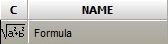In second channel row of Name column the name of the output channel created with the formula is displayed.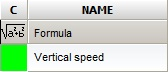## Minimum

In this section, you can change the predefined default minimum value of the graph scale.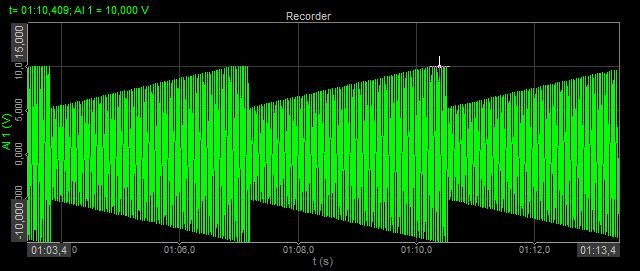## Value

In the first channel row of Value column the description of math module is displayed. For formula a symbolic record of the formula is displayed: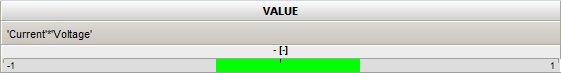for the filter, the filter settings are shown: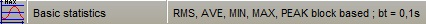In the second channel row of Value column, the live preview of the calculated value is displayed.

For the math modules with the possibility of selecting several input channels (like filters) or having several output channels for each input (like statistics), channel output section looks a little bit different - it shows one line for each output channel.

The math can also have several output channels for one input channel. The example is Statistics, where we can calculate RMS, AVE, MIN, MAX,… values for each input channel.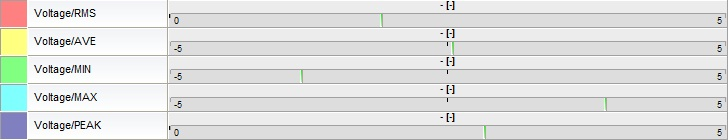If there is an error in the math module, the error caption will appear: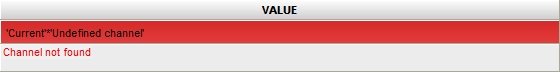There are several possible errors:

• Syntax error - the formula contains an error, for example brackets are not closed
• Circular reference error - the formula a references formula b while the formula b references formula a
• Input channel error - the input channel used in the formula has an error already (for example syntax error)

## Maximum

In this section, you can change the predefined default maximum value of the graph scale.## Unit

In the unit column, you can enter the unit of your choice, that will be displayed beside the calculated results.

## Setup

When you press the Setup button in SETUP column of Math setup screen, the window will appear depending on the selected module.

Example - Formula setup window: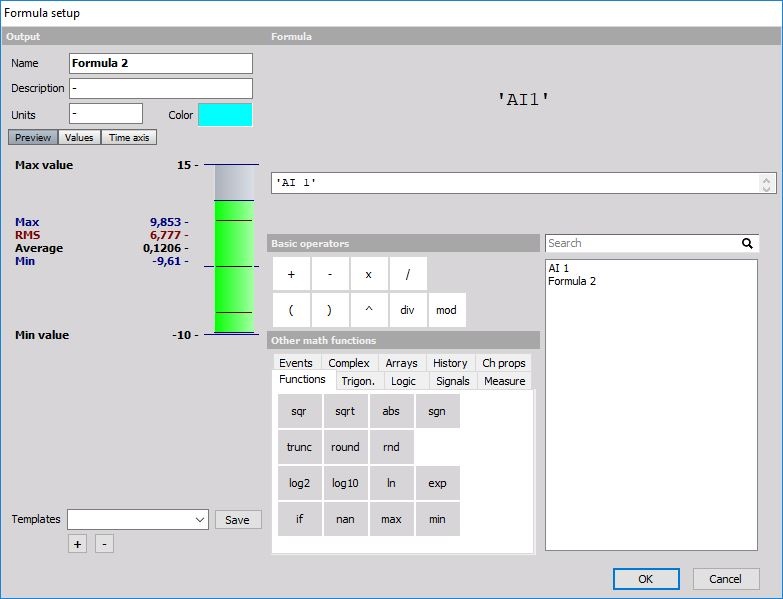Formula Setup is comprised of two sections:

1. Output: General output settings on the top left side of the window.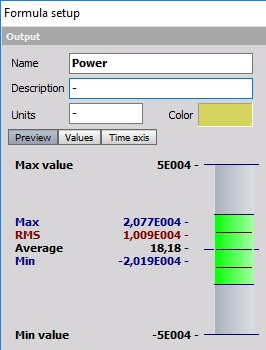1. Formula: Formula editor on the right side of the window